We think you are located in Nigeria. Is this correct?

# Test yourself now

High marks in maths are the key to your success and future plans. Test yourself and learn more on Siyavula Practice.

# Chapter 2: Rational and irrational numbers

## 2.1 Number sets

A set is a collection of objects that have something in common. A set could be a group of things that we use together, or that have similar properties. Numbers can be organised into number sets. In previous years you learnt about the following number sets:

• Natural numbers are the positive counting numbers.
• Whole numbers are the positive counting numbers plus zero.
• Integers are the whole numbers plus the negative counting numbers.

We can use set notation to write these sets using symbols:

You will learn more about set notation in later years.

number set A number set is a group of numbers that have similar properties.

natural numbers The set of natural numbers includes all the positive counting numbers.

whole numbers The set of whole numbers includes all the positive counting numbers and zero.

integers The set of integers includes all the negative counting numbers, zero, and all the positive counting numbers.

In this chapter you will learn about the set of numbers called rational numbers. A rational number is any number that can be written in the form $\frac{a}{b}$ such that $a$ and $b$ are both integers and $b\neq 0$. In set notation we write:

In set notation, the symbol $\mid$ means "such that". The symbol $\in$ means "is an element of". Therefore, $a \in \mathbb{Z}$ means " $a$ is an element of the set of integers".

Any numbers that are not part of the set of rational numbers are called irrational numbers. This set of numbers is sometimes also called the set of non-rational numbers. You will learn more about irrational numbers further along in this chapter.

rational numbers The set of rational numbers include all numbers that can be written in the form $\frac{a}{b}$ such that $a$ and $b$ are both integers and $b\neq 0$.

irrational numbers Irrational numbers are all the numbers that are not part of the set of rational numbers. The set can also be called non-rational numbers.

The following diagram shows the relationship between the number sets discussed above.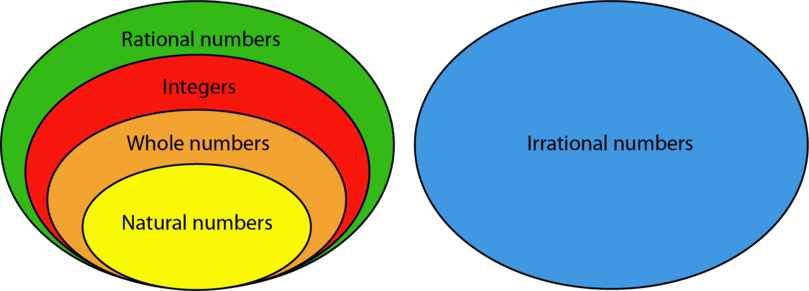## 2.2 Rational numbers

### Integers

All integers are rational numbers. This is because any integer can be written as a fraction with $1$ as the denominator. Here are some examples:

### Common fractions

All common fractions are rational numbers. In previous years you learnt that a common fraction is written in the form:

where the numerator and the denominator are both whole numbers.

In proper fractions, the numerator is smaller than the denominator. Here are some examples:

In improper fractions, the numerator is larger than the denominator. Here are some examples:

### Mixed numbers

All mixed numbers are rational numbers. In previous years you learnt that a mixed number consists of a whole number and a proper fraction. You also learnt how to write mixed numbers as improper fractions. The same method can be used for positive and negative mixed numbers. Here are some examples:

\begin{align} 2\frac{3}{4} & =\frac{11}{4}\\ 15\frac{7}{8} & = \frac{127}{8}\\ 100\frac{1}{100} & = \frac{101}{100} \\ -5\frac{3}{20}& =\frac{-103}{20}\\ -50\frac{9}{10}& =\frac{-509}{10} \end{align}

### Numbers written in decimal notation

All terminating decimals are rational numbers. In a terminating decimal, the digits that come after the decimal point come to an end. Last year you learnt how to convert numbers written in decimal notation to common fractions. Here are some examples:

\begin{array}{rcl} 0.8 & = \frac{8}{10} = & \frac{4}{5} \\ 2.5 & = \frac{25}{10} = & \frac{5}{2} \\ 0.12 & = \frac{12}{100} = & \frac{3}{25} \\ 4.08 & = \frac{408}{100} = & \frac{102}{25} \\ 0.025 & = \frac{25}{1,000} = & \frac{1}{40} \end{array}

All recurring decimals are rational numbers. In a recurring decimal, one or more decimal digits repeat in the same pattern forever. If only one or two digits repeat, we write a dot above the repeating digits. If more than two digits repeat, we write a dot above the first and last digits of the repeating pattern. Here are some examples:

\begin{align} 0.3333333 \ldots & = 0.\dot{3} \\ 0.1555555 \ldots & = 0.1\dot{5} \\ 10.27272727 \ldots & = 10.\dot{2}\dot{7} \\ 2.578578578 \ldots & = 2.\dot{5}7\dot{8} \\ 15.32656565 \ldots & = 15.32\dot{6}\dot{5} \end{align}

Recurring decimals can be written as common fractions.

terminating decimal In a terminating decimal, the digits that come after the decimal point come to an end.

recurring decimal In a recurring decimal, one or more decimal digits repeat in the same pattern forever.

### Worked example 2.1: Writing recurring decimals as common fractions (Method 1)

Write $2.\dot{1}\dot{5}$ as a common fraction.

1. Step 1: Write the number as the sum of its whole number and its decimal fraction.

2. Step 2: Write the decimal fraction as a proper fraction. The recurring digits are the numerator. The denominator has the number 9 repeated as many times as there are digits in the numerator.

The numerator must be 15. It has two digits.
The denominator must therefore be 99.

3. Step 3: Find the simplest form of the fraction from Step 2.

4. Step 4: Rewrite Step 1, but now with the decimal fraction as a proper fraction. Add the whole number and the fraction.

\begin{align} &2+\frac{5}{33} \\ =&\frac{2}{1}\times\frac{33}{33}+\frac{5}{33}\\ =&\frac{66}{33}+\frac{5}{33} \\ =&\frac{71}{33} \end{align}

This method only works if there are no non-recurring digits after the decimal point.

### Worked example 2.2: Writing recurring decimals as common fractions (Method 2)

Write $2.\dot{1}\dot{5}$ as a common fraction.

1. Step 1: Write out the recurring pattern at least three times. Let this be equal to $x$. Label the equation as (1).

2. Step 2: Multiply the written out decimal by multiples of ten. Start with 10, then try 100, then 1,000 and so on. You must get the same digits after the decimal point as those in equation (1). You must also get the repeating digits before the decimal point.

\begin{align} 10 \times 2.151515 \ldots &= 10 \times x \\ 21.51515 \ldots &= 10x \\ \end{align}

The digits after the decimal point are not the same as in equation (1), and only the number 1 of the repeating digits is before the decimal point.

Now try 100:

\begin{align} 100 \times 2.151515 \ldots &= 100 \times x \\ 215.1515 \ldots &= 100x \\ \end{align}

The digits after the decimal point are the same as in equation (1), and both recurring digits, 15, appear before the decimal point.

3. Step 3: Label the equation from Step 2 as (2). Subtract equation (1) from equation (2).

\begin{align} 2.151515 \ldots &= x \phantom{00000}(1) \\ 215.1515 \ldots &= 100x \phantom{00}(2) \end{align}

$(2)-(1)$:

\begin{align} 215.1515 \ldots - 2.151515 \ldots &= 100x - x \\ 213 &= 99x \\ \end{align}
4. Step 4: Solve the equation from Step 3.

\begin{align} 213 &= 99x \\ \frac{213}{99} &= \frac{99x}{99}\\ x&=\frac{213}{99} \\ &= \frac{71}{33} \end{align}

If there is a non-recurring digit after the decimal point, multiply equation (1) by 10 before you carry on with Step 2.

### Exercise 2.1: Write recurring decimals as common fractions

Write each of the following recurring decimals as common fractions.

1. \begin{align} &4+\frac{2}{9}\\ =&\frac{36+2}{9}\\ =&\frac{38}{9} \end{align}
2. \begin{align} &33+\frac{3}{9}\\ =&\frac{297+3}{9}\\ =&\frac{300}{9} \end{align}
3. There is a non-recurring digit after the decimal point, so you need to multiply equation (1) by 10 before you get to the values you can subtract.

You still don't have the repeating digit before the decimal point, so multiply by 100.

The repeating digits are now only the same in equations (2) and (3), so you subtract (2) from (3).

\begin{align} 100x-10x&=2.22222-0.22222 \\ 90x&=2 \\ \frac{90x}{90}&=\frac{2}{90}\\ \therefore x&=\frac{1}{45} \end{align}

### Percentages

All percentages are rational numbers. A percentage is a fraction of which the denominator is 100, and where only the numerator is written down, followed by a percentage symbol. Last year you learnt how to convert percentages to common fractions. Here are some examples:

percentage A percentage is a fraction of which the denominator is 100, and where only the numerator is written down, followed by a percentage symbol.

### Square roots

The square root of a number is a factor of the number that, when multiplied by itself, gives the number. Only square roots that have whole numbers, terminating decimals or common fractions as answers are rational numbers. Here are examples of square roots that are rational numbers:

square root The square root of a number is a factor of the number that, when multiplied by itself, gives the number.

### Exercise 2.2: Prove that numbers are rational

Prove that each of the following numbers are rational numbers.

Remember that a rational number is any number that can be written in the form $\frac{a}{b}$ such that $a$ and $b$ are both integers and $b\neq 0$.

## 2.3 Irrational numbers

Irrational numbers cannot be written in the form $\frac{a}{b}$ such that $a$ and $b$ are both integers and $b\neq 0$.

Irrational numbers do not have exact values. Examples of irrational numbers are:

• decimals that have an infinite number of decimal digits of which none are recurring, for example $2.51689753469 \ldots$
• square roots that do not have whole numbers, terminating decimals or common fractions as answers (roots), for example $\sqrt{11}$.

### Exercise 2.3: Classify numbers as rational or irrational

Classify each of the following as a rational number or a irrational number.

1. Rational number:
$\sqrt{100}=10$, and whole numbers are rational numbers.

2. Rational number:
Percentages are rational numbers.

3. Rational number:
Integers are rational numbers.

4. Irrational number:
Decimals that are not terminating or recurring are irrational numbers.

5. Rational number:
Mixed numbers are rational numbers.

6. Irrational number:
$\sqrt{27}$ does not have a whole number, terminating decimal or common fraction as its root (or answer).

7. Rational number:
Recurring decimals are rational numbers.

8. Rational number:
Integers are rational numbers.

9. Rational number:
$\sqrt{\frac{36}{121}}=\frac{6}{11}$

10. Rational number:
Terminating decimals are rational numbers.

11. Rational number:
Recurring decimals are rational numbers.

12. Irrational number:
$\sqrt{\frac{4}{20}}$ does not have a whole number, terminating decimal or common fraction as its root.

13. Irrational number:
Decimals that are not terminating or recurring are irrational numbers.

14. Irrational number:
$\sqrt{0.1}$ does not have a whole number, terminating decimal or common fraction as its root.

15. Rational number:
$-\sqrt{4}=-2$

## 2.4 Pi ( $\pi$)

Pi is an example of a irrational number. It is represented by the symbol $\pi$. It represents different ratios in a circle. You should be familiar with the following dimensions of a circle: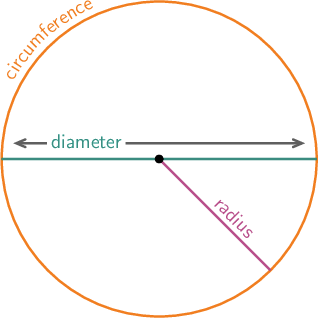For any circle, pi represents the following ratios:

Pi does not have a fixed value. To use it in calculations, we round it to 3.142 or $\frac{22}{7}$.

pi Pi $(\pi)$ is the constant ratio of the circumference to the diameter of any circle.

### Worked example 2.3: Confirming the value of $\pi$

The circle below has a circumference ( $C$) of $62.832\text{ cm}$ and an area ( $A$) of $314.16\text{ cm}^2$.

1. Calculate the ratio of the circumference to the diameter ( $d$).
2. Calculate the ratio of the area to the square of the radius ( $r$).
3. What do your answers tell you about the value of $\pi$?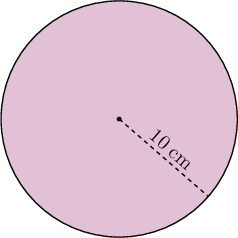1. Step 1: Calculate the ratio of the circumference to the diameter.

\begin{align} &\frac{C}{d} \\ =&\frac{62.832 \text{ cm}}{2 \times 10 \text{ cm}} \\ =&\frac{62.832 \text{ cm}}{20 \text{ cm}} \\ =&3.1416 \end{align}
2. Step 2: Calculate the ratio of the area to the square of the radius.

\begin{align} &\frac{A}{r^2} \\ =&\frac{314.16\text{ cm}^2}{10\text{ cm} \times 10\text{ cm}} \\ =&\frac{314.16\text{ cm}^2}{100\text{ cm}^2} \\ =&3.1416 \end{align}
3. Step 3: State what the answers tell you about the value of $\pi$.

Both ratios are used in calculating the value of $\pi$, and the answers are the same $-$ 3.1416. This confirms that the value of $\pi$ is close to 3.1416.

### Exercise 2.4: Calculate the value of $\pi$

For each of the following circles, calculate the value of $\pi$ to four decimal places.

1. The circle has a circumference of $25.133\;\text m$.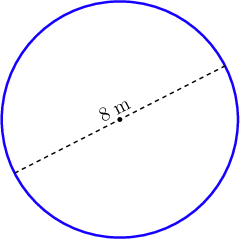\begin{align} \pi&=\frac{C}{d} \\ &=\frac{25.133\; \text m}{8\; \text m} \\ &=3.1416 \end{align}
2. The circle has an area of $12.566\text{ cm}^2$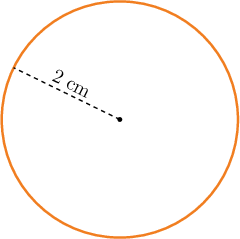\begin{align} \pi=&\frac{A}{r^2} \\ =&\frac{12.566\text{ cm}^2}{2\text{ cm} \times 2\text{ cm}} \\ =&\frac{12.566\text{ cm}^2}{4\text{ cm}^2} \\ =&3.1415 \end{align}
3. For the circle below, $C=14.14\;\text m$ and $r=2.25\;\text m$.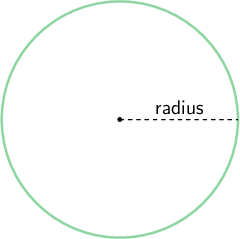\begin{align} \pi&=\frac{C}{d} \\ &=\frac{14.14\; \text m}{2\times 2.25\; \text m} \\ &=\frac{14.14\; \text m}{4.5\; \text m} \\ &=3.1422 \end{align}

## 2.5 Summary

• A number set is a group of numbers that have similar properties.
• The set of natural numbers includes all the positive counting numbers.
• The set of whole numbers includes all the positive counting numbers and zero.
• The set of integers includes all the negative counting numbers, zero and all the positive counting numbers.
• The set of rational numbers include all numbers that can be written in the form $\frac{a}{b}$ such that $a$ and $b$ are both integers and $b\neq 0$.
• The following are rational numbers:
• integers
• common fractions
• mixed numbers
• terminating decimals
• recurring decimals
• percentages
• square roots that have whole numbers, terminating decimals or common fractions as answers.
• Irrational numbers (or non-rational numbers) are all the numbers that are not part of the set of rational numbers.
• The following are irrational numbers:
• decimals that have an infinite number of decimal digits of which none are recurring
• square roots that do not have whole numbers, terminating decimals or common fractions as their roots.
• Pi ( $\pi$) is the constant ratio of the circumference to the diameter of any circle.